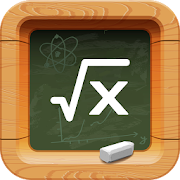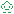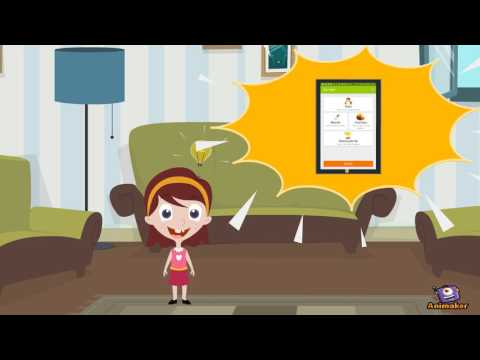# Math Tests - mathematics practice questionsEveryoneFamily Friendly
6,189
Offers in-app purchasesMath for primary and secondary school students available instantly in your device!

Improve your math fluency through thousands of multiple-choice tests. You will receive a grade at the end of each test.

You can also test yourself on the web at https://www.eductify.com/.

For 1st class/grade:
• addition and subtraction up to 100
• basic geometry 2D/3D figures and symmetry

For 2nd class/grade:
• multiplication and division
• ten based system and place value (expanded form of a number, value of digits)
• metric units of measurements (units of length, weight, volume, area - meters, km, liters, tons)
• US standard units of measures (cups-c, pints-pt, fl oz, yards-yd,inches-in, feet-ft,ounces, pounds-lb)
• time (minutes, hours, seconds, days, weeks), converting am/pm time to 24 hour time

For 3rd class/grade
• long subtraction and addition in columns with or without carrying, multiplication, division, divisibility, operations with brackets, parentheses and braces
• rounding of numbers
• Roman numerals and the Greek alphabet
• ten based system and place value (expanded form, the value of digits)

For 4th class/grade:
• fraction practice (numerator and denominator, all operations, compare with whole numbers, mixed numbers, complex fractions, fractions in the lowest term, adding and subtracting fractions, multiplying fractions, dividing fractions)
• rounding to the nearest ten, hundred or thousand
• decimals (decimals and fractions, compare with fractions, multiplying and dividing decimals)

App also includes:
• divisibility (odd and even numbers, divisibility by 3, 4, 5, 6, 8, 9, 10)
• arithmetic
• negative numbers and absolute value (compare negative numbers, add and subtract negative numbers, multiplying and dividing negative numbers, adding and subtracting absolute value)
• equations with variables (one-step equations, equations with negative numbers, quadratic equations, solving equations with brackets)
• inequalities and intervals (inequalities with all operations and negative numbers)
• geometry (number of sides, faces and vertices, area of squares, perimeter of triangles, trapezoids and rhomboids, altitudes and medians, volume of prisms, pyramids, cones, spheres, perimeters)
• square roots, powers and exponents (roots and powers with the negative base, negative exponent, fractions as exponents, numerical and algebraic expressions with exponents, scientific notation)
• basic algebra
• sets, set operations, Venn diagrams and types of numbers
• percents %, ratios, cross-multiplication word problems, the rule of three, direct and indirect variation
• angles and their types (right, obtuse, acute; adjacent, vertical, linear pair, alternate, corresponding, consecutive), angles at the center and circumference
• linear functions, range and domain
• algebraic expressions

More will be added soon since our aim is to cover the complete curriculum for Primary and High school. This app is suitable not only for children but also for older students and adults.

Results are tracked. You can review your mistakes and progress. The app works offline as well!

This app is like having dozens of math worksheets and exercises for 1st, 2nd, 3rd, 4th, 5th, 6th and 7th grade/class directly in your device with results and solutions immediately available. Since it works offline you learn to solve math and algebra problems whenever you want! The exercises are ideal for better math fluency and are suitable for kids and adults as well.

Key features overview:
• dozens of math categories and subcategories
• thousands of questions
• each test is evaluated - you are given a grade like in school
• test history allows you to see where you made mistakes
• statistics showing your progress over time
• includes a math championship where you can compete with other users to learn math in a fun way
Read more
Collapse

Review Policy
4.6
6,189 total
5
4
3
2
1
Loading...

## What's New

* Support for tablet 10'' screen size
* Squirrel Eduard will help you with learning
* New subject English Grammar Tests (for non native speakers)
* New questions
* Minor improvements and bug fixes
Read more
Collapse

## Additional Information

Updated
March 6, 2019
Size
18M
Installs
500,000+
Current Version
1.5.3
Requires Android
4.1 and up
Content Rating
Everyone
Interactive Elements
Digital Purchases
In-app Products
\$0.99 - \$16.95 per item
Permissions
Offered By
Holucent
Developer
Gorkeho 52, Brno, Czech Republic
©2019 GoogleSite Terms of ServicePrivacyDevelopersArtistsAbout Google|Location: United StatesLanguage: English (United States)
By purchasing this item, you are transacting with Google Payments and agreeing to the Google Payments Terms of Service and Privacy Notice.